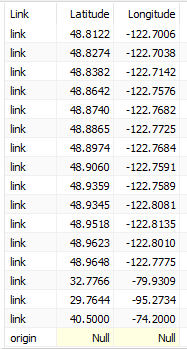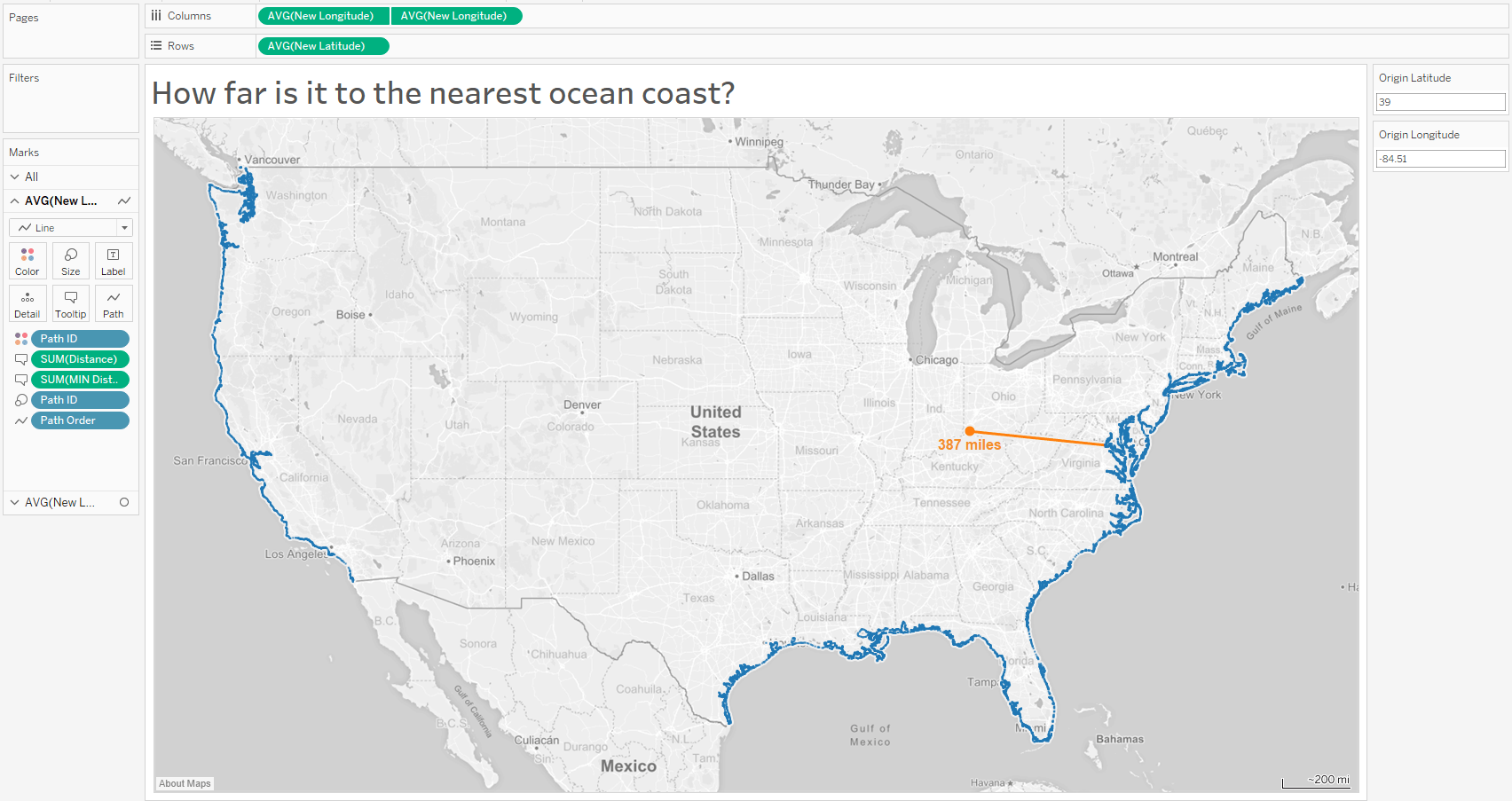## Data + Science

[ad_1]

2/20/2018
Finding the Nearest Ocean Coast or any Nearest Point on a Map in Tableau

Brooke asked on Twitter if there was a way to find the nearest ocean coast for a given point on a map. I mocked up a solution for this and posted it on Twitter here. In this tutorial, I will walk through the steps of my mock up.### How to find the nearest coastal point

Step 1: Build the coastal points (or use any set of points with latitude and longitude)

Any data set of points with latitude and longitude could be used, but to get the coasts for the mock up, I started with a data set of the continental United States (USA2163) in polygon format (latitude, longitude, polygon ID, Point Order). I plotted the points and filtered out the points that are not on the coast. This was pretty easy, just highlighting the Mexican and Canadian borders and the Great Lakes and then excluded them.

Once this was filtered out, I exported the data set with only those points into a new file. If you prefer to use your own file of points then all you need is latitude, longitude and some sort of Point ID.

Step 2: Add an empty row to the data and import into Tableau

I added an empty row to the data. This could be done by joining a single row of data up with the polygon points, but I just manually added one row before importing into Tableau. This empty row will be the point of origin on the map. I also created a new field called “Link”. The destination points should be marked as “Link” (or Destination) and the empty row is marked “origin”. If you want to set the origin point in the data, then add a field for “Origin Latitude” and “Origin Longitude” and enter that data. Otherwise, you can create these fields in Tableau or use a Parameter, which is what I did in the mock up.

The data is now ready to be import into Tableau.Step 3: Create parameters and calculated fields

Parameter Name: Origin Latitude as a Float (optional)
Parameter Name: Origin Longitude as a Float (optional)

Calculated Field Name: Origin Latitude
Formula: [Parameters].[Origin Latitude]
(this could also be hardcoded or taken from a data source)

Calculated Field Name: Origin Longitude
Formula: [Parameters].[Origin Longitude]
(this could also be hardcoded or taken from a data source)

In order to calculate the closest point to the origin, we need to calculate the distance. I used the following distance formula in Tableau to calculate the distance between the origin point and all of the other points.

Calculated Field Name: Distance
Formula:
3959 * ACOS(SIN(RADIANS([Origin Latitude])) * SIN(RADIANS([Latitude])) + COS(RADIANS([Origin Latitude]))
* COS(RADIANS([Latitude])) * COS(RADIANS([Longitude]) – RADIANS([Origin Longitude])))

Next, create a calculated field to get the minimum distance in the data set.

Calculated Field Name: MIN Distance
Formula: {fixed : MIN([Distance])}

If you are using a Parameter to change the lat/long then calculate a new set of lat/long that will adjust with the parameter for the origin point. I included additional elseif lines to populate the lat/long for the City dropdown menu.

Calculated Field Name: New Latitude
Formula:
if [Link] = ‘link’ then [Latitude]
else [Parameters].[Origin Latitude]
END

Calculated Field Name: New Longitude
Formula:
if [Link] = ‘link’ then [Longitude]
else [Parameters].[Origin Longitude]
END

Finally, create two fields for the path, Path ID to group the points together and Path Order to specify the other of the path for the line.

Calculated Field Name: Path ID
Formula:
If [Distance] = [MIN Distance] then 1
elseif [Link] = ‘origin’ then 1
else NULL
END

Calculated Field Name: Path Order
Formula:
If [Distance] = [MIN Distance] then 1
elseif [Link] = ‘origin’ then 2
else NULL
END

As an optional step, create a label for the distance for the origin point.

Calculated Field Name: Label
Formula:
if [Link] = ‘origin’ then [MIN Distance]
else NULL
END

Step 4: Building the Viz

Move New Longitude to Columns
Move New Latitude to Rows
Move Point ID to Detail (In the coastal file I used Point Order and Sub Polygon ID)
Move Path ID to Size (Optional – This will size the origin point larger than the other points)

You should now have a map with the points plotted. In the mock up this is all of the coastal points.Duplicate New Latitude on Columns.

On the second Marks Card:

Remove everything from Detail
Move Path ID to Color
Change Mark type to Line
Move Path Order to Path
Move MIN Distance to Tooltip
Move Label to Label
Right-click on the duplicated New Latitude and select Dual AxisGive it a try in the viz below. Select a City from the dropdown menu or enter your own latitude and longitude within the continental U.S. The viz will update, calculating and plotting the minimum distance to the nearest ocean coast. Feel free to download the Tableau Public workbook for the data and viz.

I hope you find this information useful. If you have any questions feel free to email me at Jeff@DataPlusScience.com

Jeffrey A. Shaffer

Follow on Twitter @HighVizAbility

Read More …

[ad_2]